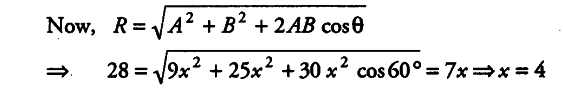# Two forces whose magnitudes

Two forces whose magnitudes are in the ratio 3 : 5 give a resultant of 28 N. If the angle of their inclination is 60° Find the magnitude of each force.

Let A and B be the two forces.
Then, A = 3x, B = 5x, R = 28 N and \theta = 60°
Thus, A/B = 3/5.’.Forces are A = 12 N B = 20 N